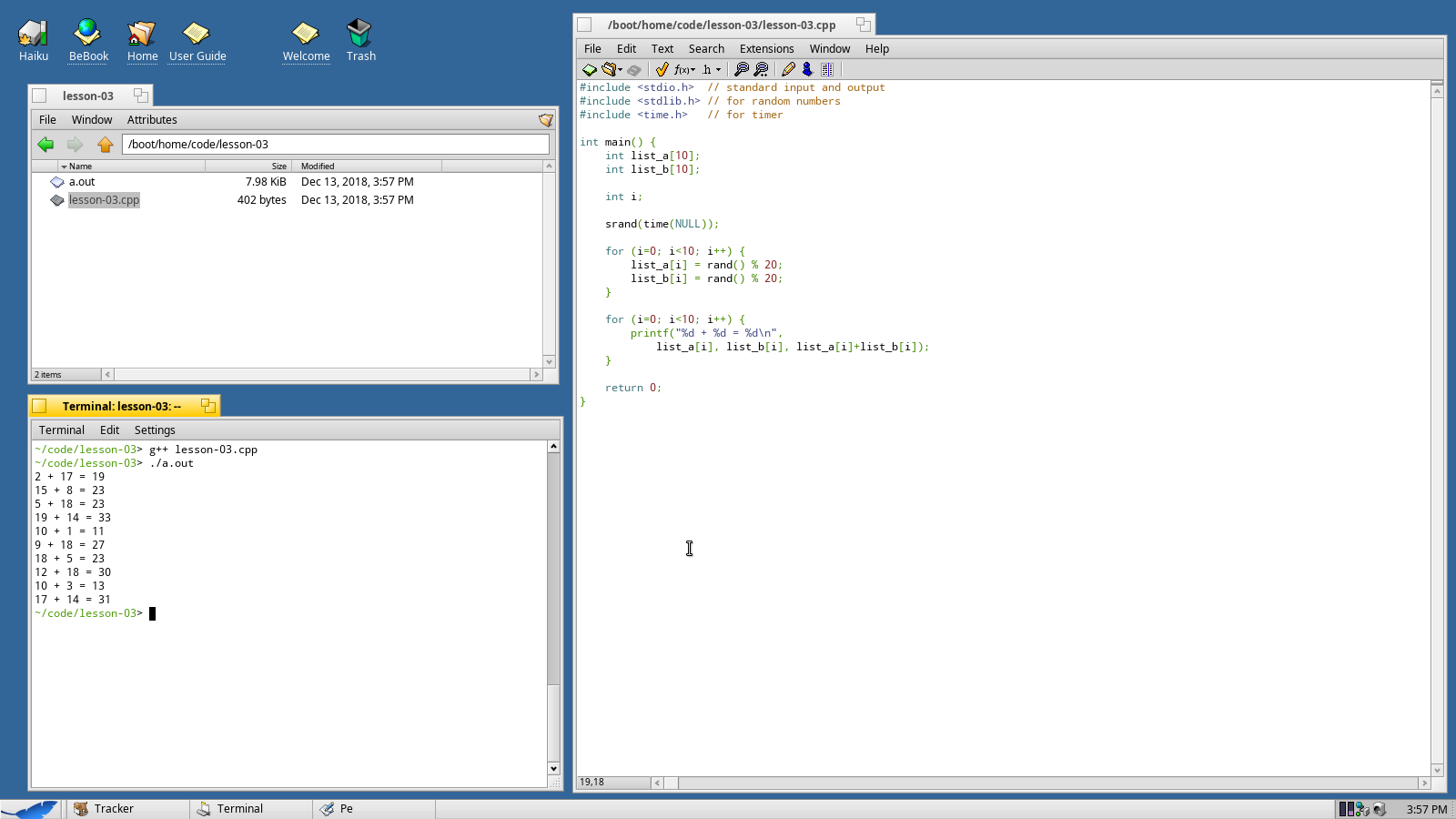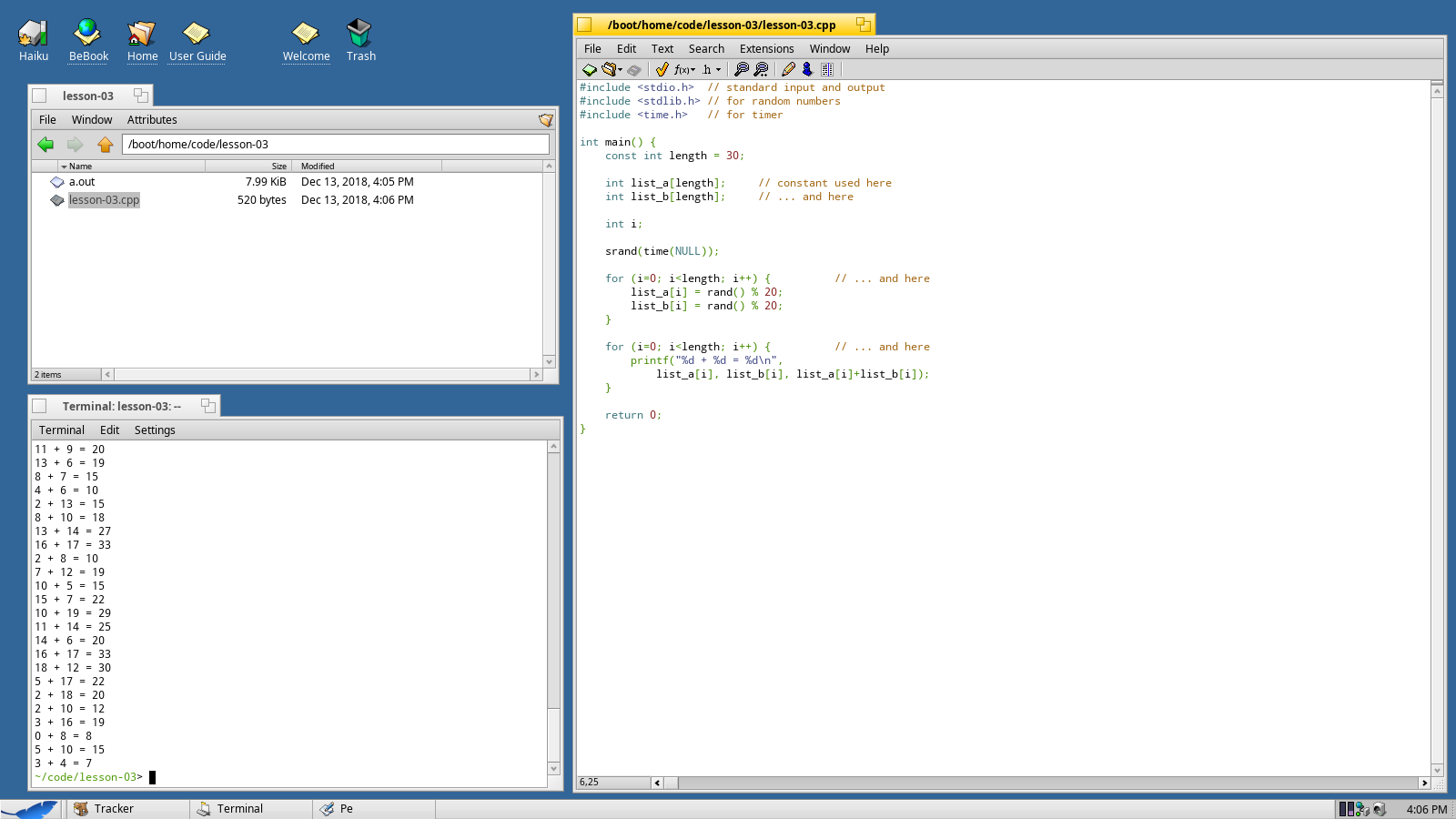Web Developer

# Lesson 3 - Arrays and Loops

Arrays allow you to store a list of items and loops allow you to step through the list and perform actions on each one. In this lesson we'll be creating two arrays of integers and then adding each of pair and outputting the results.

Along the way we're going to learn two more important concepts: constants and initialization.

First, let's get our basic main function started. We'll be needing two additional libraries, `stdlib.h` and `time.h`.

``````#include <stdio.h>    // standard input and output
#include <stdlib.h>    // for random numbers
#include <time.h>    // for timer

int main() {
return 0;
}``````

## Defining Storage

Before we add together two lists of numbers, we're going to need to create two lists of numbers.

Inside the main function, write:

``````int list_a;
int list_b;``````

This will create two arrays of integers. Each array will be 10 items long.

In order to use a loop to go through the list, we'll need an iterator - a number that's just used for counting up.

``int i;``

## Generating Random Numbers

Before we can start filling the list with random numbers we need to set up the random number generator.

When we start up the random number generator, we want to hand it a different number each time - so we're going to use the current date and time (since the current date and time should be different every time you run the program).

``srand(time(NULL));``

Now we're going to set up our first loop.

``````for (i=0; i<10; i++) {

}``````

Let's take a look at this piece by piece so that you can understand what's going on here. The basic structure is that the `for` command will use the rules `(i=0;i<10;i++)` and each time through the loop it will do whatever is in between the `{ }` curly braces.

The first rule `i=0` tells the `for` command how to start the loop. The second rule `i<10` tells the `for` command when to stop the loop. The third rule `i++` tells the `for` command what to do at the end of each loop (in this case, increase `i` by 1).

It's also important to note that we are going to go from 0 to 9, not 1 to 10. Arrays in C/C++ are zero-indexed - the first item in `list_a` is `list_a`. The 10th item in `list_a` is `list_a`.

We'll now fill in the loop by assigning a random number for each pass.

``````for (i=0; i<10; i++) {
list_a[i] = rand() % 20;
list_b[i] = rand() % 20;
}``````

The `rand()` function is pretty straightforward - it just returns a random integer. You'll notice a new operator `%`, called the modulus operator. This operator will divide one number by another and then tell you the remainder.

For example, the answer of 25 divided by 20 is 1 remainder 5. Therefore, the result of `25 % 20` is 5.

If you think back to your 4th math you'll remember that clearly the remainder can never be larger than the divisor - which gives us a quick and easy way to take a random number (which could be anything, really) and turn it into a random number between 0 and 19.

The last thing we need to do in this program is to iterate through the list and add the number pairs together. This is pretty straightforward: we're going to make a loop like the one above and do the addition inside the loop.

``````for (i=0; i<10; i++) {
printf("%d + %d = %d\n",
list_a[i], list_b[i], list_a[i]+list_b[i]);
}``````

You can see that we told `printf` that we're printing some text that will contain three numbers, then we specify those numbers as `list_a[i]`, `list_b[i]`, and `list_a[i]+list_b[i]`.

C/C++ is smart enough to do the addition first and then just pass the result along to `printf` so that you don't need to store it as a separate variable.You should be able to compile and run your program now and see some results.

## Improving the Program With Constants

Sometimes you want to store a value, but you also want to ensure that that value won't get changed by mistake. Use the `const` directive when creating a variable to make it a constant.

``const int length;``

However, since we can't change the value of a constant, that means we have to initialize the constant when we create it.

``const int length = 10;``

From here on out, instead of using `10` in our loops, we'll use `length`. If we decide that we want to make a list of 100 numbers instead of 10 we will only have to change length, we won't have to change our `for` loops.

You can also use a constant when setting the length of an array. You cannot set the length of an array with an ordinary variable.Full source code below:

``````#include <stdio.h>    // standard input and output
#include <stdlib.h>    // for random numbers
#include <time.h>    // for timer

int main() {
const int length = 10;

int list_a[length];            // constant used here
int list_b[length];            // ... and here

int i;

srand(time(NULL));

for (i=0; i<length; i++) {        // ... and here
list_a[i] = rand() % 20;
list_b[i] = rand() % 20;
}

for (i=0; i<length; i++) {        // ... and here
printf("%d + %d = %d\n",
list_a[i], list_b[i], list_a[i]+list_b[i]);
}

return 0;
}``````

## Challenge

Now that you've hopefully followed along for three lessons, here's a little homework.

Using a constant, modify the above program to add 30 random numbers between 0 and 99.